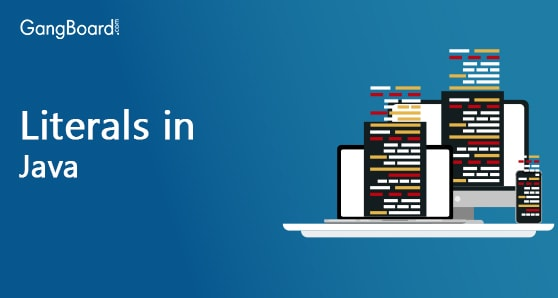Special Offer - Enroll Now and Get 2 Course at ₹25000/- Only Explore Now!# Literals in Java

May 1st, 2019

## Literals in Java

Any type of data can be used inside java programming. These data can be accessed using or without using variables inside our program.
Now, we’ll discuss that how these data is been divided and how many categories of data we have totally.

#### There are 5 basic categories which are:

1. Integral Literals
2. Floating Literals
3. Character Literals
4. String Literals
5. Boolean Literals

### Integral Literals :

Integrals are nothing but the whole numbers as we say in mathematics (i.e, the numbers without decimals and fractions).
This integral literal can be a binary number (base 2) or an octal (base 8) or a decimal (base 10) or a hexadecimal (base 16).
Now, these integrals are divided into the following types:

• byte
• short
• int
• long

The value for these integral type variables can be represented as decimals, octals, hexadecimals and binaries, which are also known as Decimal Literals, Octal Literals, Hexadecimal Literals and Binary Literals (which is added from jdk 1.7). Each of these above mentioned types (byte, short, int, long) has their own specific range of values, which we’ve already seen under the topic ‘Data Types’. Which means it accepts values only within its own range.
If any value beyond its range is tried to be assigned, then we will end up with loss of data. Therefore, while using decimals, octals and hexadecimals also if the value accommodates within its given specific range, then that value will be accepted by those literals while assigning.
Now, let us have a glance at the following :

#### Decimal Literals :

Allows digits from 0 – 9. e.g. int a = 123;

#### Octal Literals :

Allows digits from 0 – 7. But, these values should be prefixed with ‘0’. e.g. int a = 0147;

Allows digits from 0 – 9 and alphabets from a – f. And must be prefixed with ‘0x’ or ‘0X’. Supports uppercase and lowercase. Even though java is case-sensitive, here it is exceptional. e.g.int a = 0x253Face;

#### Binary Literals :

From jdk 1.7, we can have values of literals in binary form also. It allows only two digits in its values i.e, 0 and 1. But, these values have to be prefixed with ‘0b’ or ‘0B’.
Example: int a = 0b1011;

#### Example:

 ```public class Literals { public static void main(String[] args) { int a = 123;                  // Decimal Form int b = 0147;               // Octal Form int c = 0x253Face;      // Hexadecimal Form int d = 0b1011;                       // Binary Form System.out.println("Decimal Form : "+a); System.out.println("Octal Form : "+b); System.out.println("Hexadecimal Form : "+c); System.out.println("Binary Form : "+d); } }```

#### Output :

 ```Decimal Form : 123 Octal Form : 103 Hexadecimal Form : 39058126 Binary Form : 11```

### Floating Literals :

Floating literals are nothing but integrals values with a fractional part. We can’t provide octal, hexadecimal and binary values as floating literals. Only decimal values are allowed.

#### Example:

 5.3866e4 -> 53, 866 3e-11 -> 00000000003 1e+4 -> 10000 7.341E-3 -> 0.007341 3.5E+4 -> 35000 0.4e-6 -> 0.0000004 5.e10-> 50000000000

### Character Literals :

Alphabets or any values provided within single quotes are Character Literals.
Character Literals are of 4 types :

• Using Single Quotes
• Using Integral Literal
• Unicode Representation
• Escape Sequence

#### Using Single Quotes

e.g. char c = ‘l’;

#### Using Integral Literal

We can assign Unicode value.

##### Example:

char c = 052;
which represents character *
Here, the allowed range is 0 to 65535.

#### Unicode Representation

i.e, Representation of Unicode values in Hexadecimal pattern.

##### Example:

char c = ‘\u0062’; // represents lowercase character b
or
char c = ‘\u0041’; // represents uppercase character A

#### Escape Sequence

##### Example:
 ```char c = ‘\n’; or char c = ‘\t’; or char c = ‘\b’;```

### String Literals :

String Literals can be represented as a sequence of characters within double quotes.
String s = “New Delhi”;
String stmt = “Qutub Minar is in New Delhi,” + “ which is the capital of India.”;

### Boolean Literals :

Boolean literals contains only two values. i.e, true and false [Since, java is case sensitive, lowercase ‘true’ and ‘false’ are only allowed as boolean literals]. None of any other values are allowed here.
Example:

 ```boolean a=true; boolean b=false; boolean a=0; boolean b=1; boolean a= TRUE; boolean b=FALSE; boolean a=True; boolean b=False;```